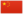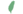# 风皮

Breezepelt小风 Breezekit  小風非官
 风爪 Breezepaw  風掌
 风皮 Breezepelt  風皮

Q2602：风族公猫，自《预视力量》登场的角色

## 细节

### 外貌

• 他是一只身形精瘦、:1琥珀色眼睛的黑色公猫。:137

## 登场书目

• 预视力量 (Q152)[配角][首次登场🐱]
• 暗河汹涌 (Q153)[配角]
• 驱逐之战 (Q154)[主要角色]
• 天蚀遮月 (Q155)[配角]
• 暗夜长影 (Q156)[对话提及]
• 拂晓之光 (Q157)[配角]
• 第四学徒 (Q158)[配角]
• 战声渐近 (Q159)[配角]
• 暗夜密语 (Q160)[配角]
• 月光印记 (Q161)[配角]
• 武士归来 (Q162)[配角]
• 群星之战 (Q163)[配角]
• 学徒探索 (Q170)[猫物闲角]
• 雷影交加 (Q171)[配角]
• 天空破碎 (Q172)[猫物闲角]
• 极夜无光 (Q173)[猫物闲角]
• 烈焰焚河 (Q174)[配角]
• 风暴来袭 (Q175)[配角]
• 迷失群星 (Q3563)[配角]
• 静默冰融 (Q3579)[配角]
• 叠影重障 (Q3624)[配角]
• 暗由心生 (Q4183)[配角]
• 无星之地 (Q4385)[配角]
• 迷雾明光 (Q4621)[配角]
• 河翻浪涌 (Q4643)[猫物闲角]
• 天穹将倾 (Q4799)[猫物闲角]
• 黑莓星的风暴 (Q182)[猫物闲角]
• 虎心的阴影 (Q185)[猫物闲角]
• 鸦羽的拷问 (Q186)[主要角色]
• 松鼠飞的希冀 (Q3561)[配角]
• 灰条的誓言 (Q4057)[猫物闲角]
• 一星的告解 (Q4826)[配角]
• 冬青叶的故事 (Q187)[猫物闲角]
• 雾星的征兆 (Q188)[猫物闲角]
• 鸽翅的沉默 (Q192)[配角]
• 褐皮的族群 (Q3566)[猫物闲角]
• 黛西的至亲 (Q3629)[配角]
• 斑毛的反抗 (Q4337)[配角]
• 族群的猫 (Q200)[配角]
• 终极指南 (Q204)[配角]

## 参考文献

这篇文章基于CC BY-SA 3.0许可使用了猫武士维基（英语）Breezepelt一文中的部分内容。
1. 在同一份由维琪授意、Su所公布的黑森林名单中，官网家谱已将雪暴翅、亮花和沼云标注为星族成员，短尾标注为黑森林成员；鹿脚、百合须和缠果的死后去处则暂时没有更进一步的资讯。